# Prime NumbersPage 1

#### WATCH ALL SLIDES

Slide 1Prime Numbers

Some Simple Tips and Reminders

Slide 2## Definitions

Even Numbers – Any number that can be divided by 2

Odd Numbers – Any number that cannot be divided by 2

Composite Number – An integer that can be divided by at least one other number (a factor) other than itself

Slide 3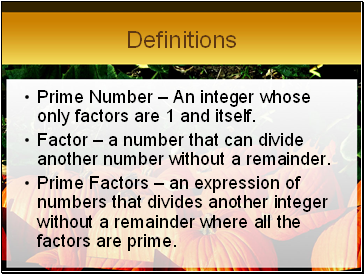Definitions

Prime Number – An integer whose only factors are 1 and itself.

Factor – a number that can divide another number without a remainder.

Prime Factors – an expression of numbers that divides another integer without a remainder where all the factors are prime.

Slide 4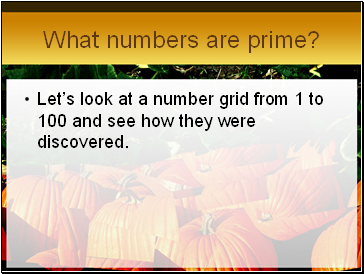## What numbers are prime?

Let’s look at a number grid from 1 to 100 and see how they were discovered.

Slide 5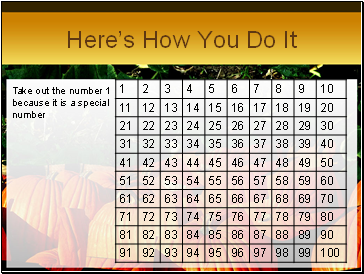## Here’s How You Do It

Take out the number 1 because it is a special number

Slide 6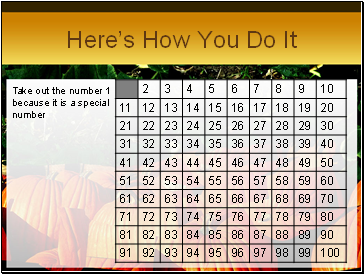Here’s How You Do It

Take out the number 1 because it is a special number

Slide 7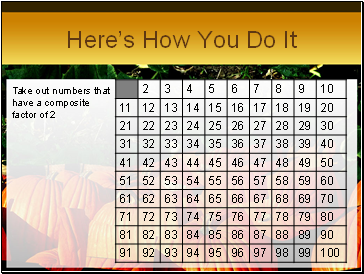Here’s How You Do It

Take out numbers that have a composite factor of 2

Slide 8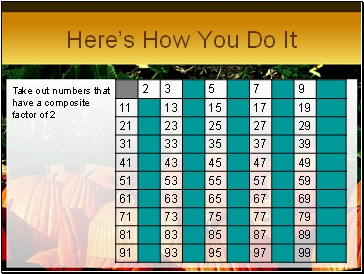Here’s How You Do It

Take out numbers that have a composite factor of 2

Slide 9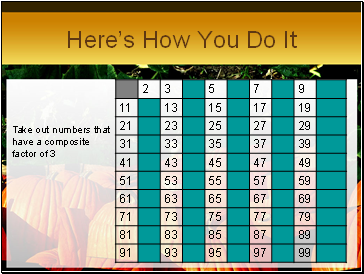Here’s How You Do It

Take out numbers that have a composite factor of 3

Slide 10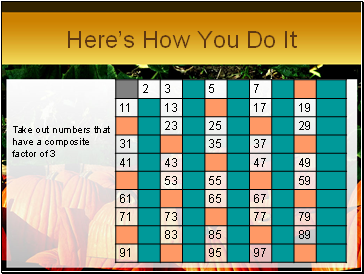Here’s How You Do It

Take out numbers that have a composite factor of 3

Slide 11Here’s How You Do It

Take out numbers that have a composite factor of 5

Slide 12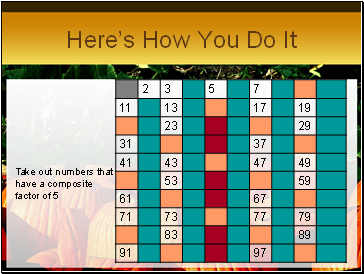Here’s How You Do It

Take out numbers that have a composite factor of 5

Slide 13Here’s How You Do It

Take out numbers that have a composite factor of 7

Slide 14## The PRIME Numbers!

Take out numbers that have a composite factor of 7

Slide 15Go to page:
1  2